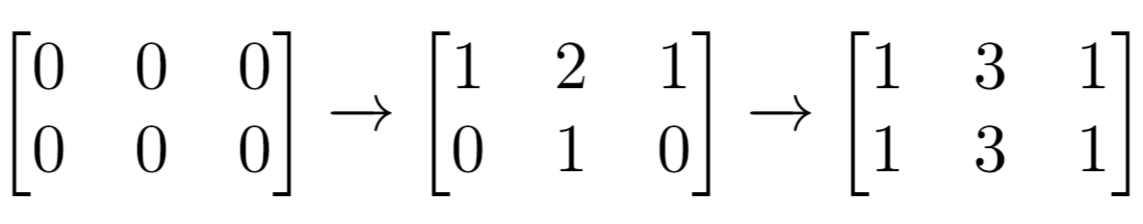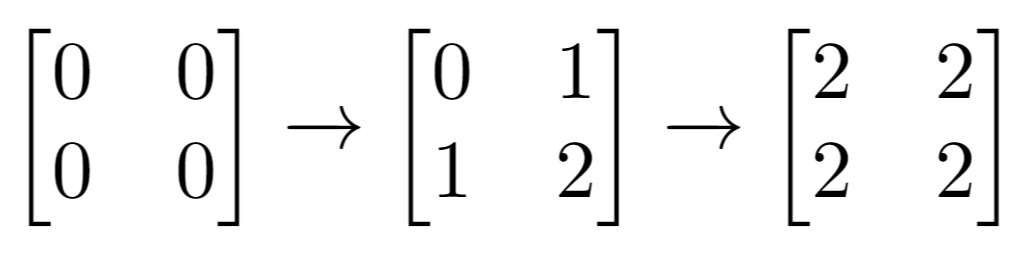# LeetCode-1252. Cells with Odd Values in a Matrix

Given n and m which are the dimensions of a matrix initialized by zeros and given an array indices where indices[i] = [ri, ci]. For each pair of [ri, ci] you have to increment all cells in row ri and column ci by 1.

Return the number of cells with odd values in the matrix after applying the increment to all indices.

Example 1:Input: n = 2, m = 3, indices = [[0,1],[1,1]]
Output: 6
Explanation: Initial matrix = [[0,0,0],[0,0,0]].
After applying first increment it becomes [[1,2,1],[0,1,0]].
The final matrix will be [[1,3,1],[1,3,1]] which contains 6 odd numbers.


Example 2:Input: n = 2, m = 2, indices = [[1,1],[0,0]]
Output: 0
Explanation: Final matrix = [[2,2],[2,2]]. There is no odd number in the final matrix.


Constraints:

• 1 <= n <= 50
• 1 <= m <= 50
• 1 <= indices.length <= 100
• 0 <= indices[i] < n
• 0 <= indices[i] < m

题解：

class Solution {
public:
void add(int x, int y, int n, int m, vector<vector<int>> &mat) {
for (int i = 0; i < m; i++) {
mat[x][i]++;
}
for (int i = 0; i < n; i++) {
mat[i][y]++;
}
}
int oddCells(int n, int m, vector<vector<int>>& indices) {
vector<vector<int>> mat(n, vector<int>(m, 0));
for (int i = 0; i < indices.size(); i++) {
}
int res = 0;
for (int i = 0; i < n; i++) {
for (int j = 0; j < m; j++) {
if (mat[i][j] % 2 != 0) {
res++;
}
}
}
return res;
}
};

11-1068204-0354
11-13166
04-23597
03-21465
09-2241
01-2416
05-24157
02-02125
03-0632
08-0964
11-1053
08-1336
03-05114
07-0348
11-1762
11-1180
12-0285